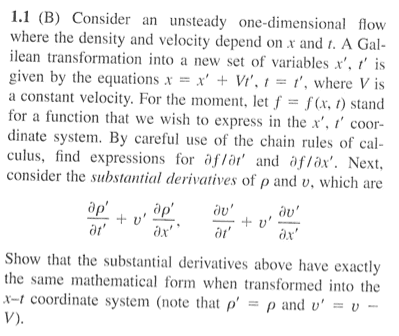# Show Derivative of Function Takes Same Form as

## Homework StatementChain Rule

## The Attempt at a Solution

So we have that $f = f(x,t)$ as well as the transformations $x = x' + Vt'$ and $t = t'$

By the chain rule:

$$\frac{\partial{f}}{\partial{t'}} = \frac{\partial{f}}{\partial{x}}\frac{\partial{x}}{\partial{t'}} + \frac{\partial{f}}{\partial{t}}\frac{\partial{t}}{\partial{t'}}$$

$$\Rightarrow \frac{\partial{f}}{\partial{t'}} = \frac{\partial{f}}{\partial{x}} * \left [ \frac{\partial{x}}{\partial{t'}}+V+t'\frac{\partial{V}}{\partial{t'}}\right ] + \frac{\partial{f}}{\partial{t}}\frac{\partial{t'}}{\partial{t'}}$$

$$\Rightarrow \frac{\partial{f}}{\partial{t'}} = \frac{\partial{f}}{\partial{x}} * \left [ \frac{\partial{x}}{\partial{t'}}+V+t'\frac{\partial{V}}{\partial{t'}}\right ] + \frac{\partial{f}}{\partial{t}}$$

Not really sure what the next move is? Is the above equation in its simplest form? Or can I do something more with it?

Also, I don't really see how I go about transforming $\rho'$ and $v'$ into the x-t coordinates?

Last edited:

## Answers and Replies

Homework Helper
Note that there are only partial derivatives.
So then isn't
$$\frac{\partial x}{\partial t'} = V$$?

Homework Helper
You made a mistake:
$$\frac{\partial f}{\partial t'}=V\frac{\partial f}{\partial x}+\frac{\partial f}{\partial t}$$
as:
$$\frac{\partial x}{\partial t'}=V\quad\frac{\partial t}{\partial t'}=1$$

Last edited:
Gold Member
You made a mistake:
$$\frac{\partial f}{\partial t'}=V\frac{\partial f}{x}+\frac{\partial f}{\partial t}$$
as:
$$\frac{\partial x}{\partial t'}=V\quad\frac{\partial t}{\partial t'}=1$$

Shouldn't that be $$\frac{\partial f}{\partial x}$$?

Homework Helper
Hi Saladsamurai!(have a rho: ρ and a curly d: ∂)
Not really sure what the next move is? Is the above equation in its simplest form? Or can I do something more with it?

Now you put in ∂x'/∂t etc, which you can read off the Galilean transformation.Also, I don't really see how I go about transforming $\rho'$ and $v'$ into the x-t coordinates?

But the question tells you that … ρ' = ρ, v' = v - V.